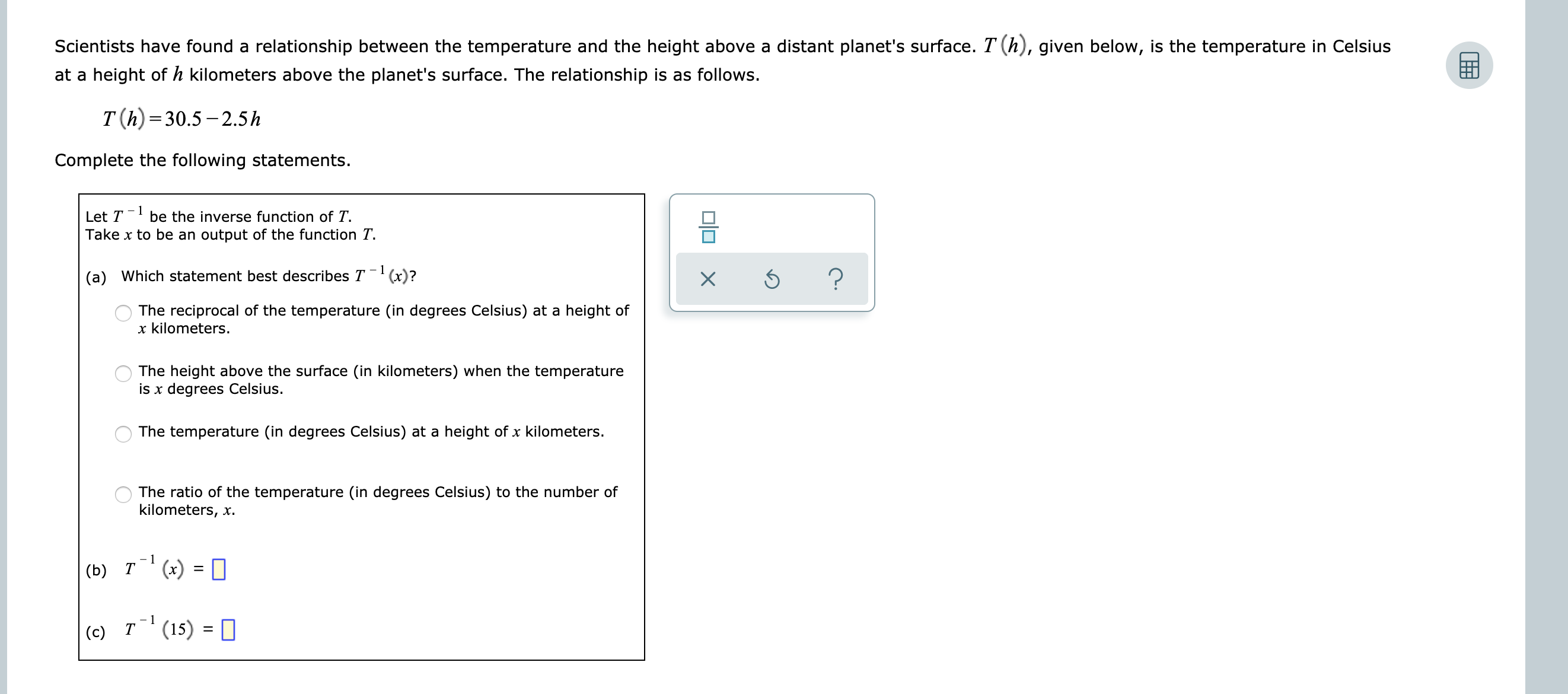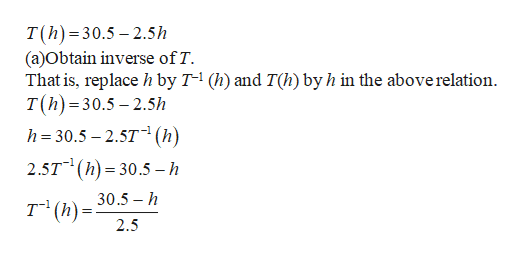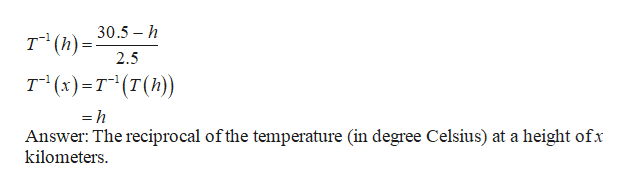# Scientists have found a relationship between the temperature and the height above a distant planet's surface. T (h), given below, is the temperature in Celsiusat a height ofh kilometers above the planet's surface. The relationship is as follows.T (h) 30.5-2.5hComplete the following statements.- 1Let Tbe the inverse function of TTake x to be an output of the function T?(a) Which statement best describes T(x)?XThe reciprocal of the temperature (in degrees Celsius) at a height ofx kilometers.The height above the surface (in kilometers) when the temperatureis x degrees Celsius.The temperature (in degrees Celsius) at a height of x kilometers.The ratio of the temperature (in degrees Celsius) to the number ofkilometers, x- 1(x) =(b) Т- 1Т(15)(c)OO

Question
203 views

see attachmenthelp_outlineImage TranscriptioncloseScientists have found a relationship between the temperature and the height above a distant planet's surface. T (h), given below, is the temperature in Celsius at a height ofh kilometers above the planet's surface. The relationship is as follows. T (h) 30.5-2.5h Complete the following statements. - 1 Let T be the inverse function of T Take x to be an output of the function T ? (a) Which statement best describes T(x)? X The reciprocal of the temperature (in degrees Celsius) at a height of x kilometers. The height above the surface (in kilometers) when the temperature is x degrees Celsius. The temperature (in degrees Celsius) at a height of x kilometers. The ratio of the temperature (in degrees Celsius) to the number of kilometers, x - 1 (x) = (b) Т - 1 Т (15) (c) OO fullscreen
check_circle

Step 1

The relation between the temperature and the height above a distance planet is,help_outlineImage TranscriptioncloseT(h) 30.5-2.5h (a)Obtain inverse of T That is, replace h by T-1 (h) and T(h) by h in the above relation T(h) 30.5-2.5h h 30.5 2.5T (h) 2.5T h 30.5 - h 30.5 h т"(): 2.5 fullscreen
Step 2

(b) Given that x  is the output for the temperature T .

Obtai...help_outlineImage Transcriptionclose30.5 h т" (): 2.5 т (:)-т"(т(h) =h Answer: The reciprocal ofthe temperature (in degree Celsius) at a height ofx kilometers fullscreen

### Want to see the full answer?

See Solution

#### Want to see this answer and more?

Solutions are written by subject experts who are available 24/7. Questions are typically answered within 1 hour.*

See Solution
*Response times may vary by subject and question.
Tagged in

### Other